Study S4 Physics Sound - Geniebook# Sound

In today’s article we will learn about Sound. We will cover:

• How is sound generated?
• What is the mechanism by which sound travels?
• Why does sound need a medium for transmission?
• How speed of sound differs in solids, liquids, and gases?

## What Is Sound ?

Sound is a form of energy that is transferred from one point to another as a longitudinal wave.

There are two main kinds of waves:

• Transverse Waves: The wave in which the wave particles vibrate in a direction perpendicular to the direction of flow of the waves is called transverse waves.
• Longitudinal Waves: The waves in which the wave particles vibrate in the direction parallel to the direction of the flow of waves are called longitudinal waves.

Sound waves are transmitted only as longitudinal waves.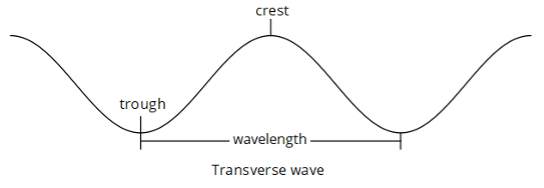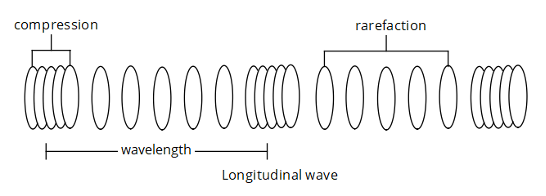### How Is Sound Produced ?

Sound is produced by vibrating sources placed in a medium.

### How Do Sound Waves Propagate ?

Sound waves propagate as a series of compressions and rarefactions.

• Compressions are regions where the medium’s density or pressure is higher than the surrounding region.
• Rarefactions are regions where the medium’s density or pressure is lower than the surrounding region.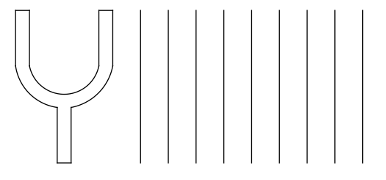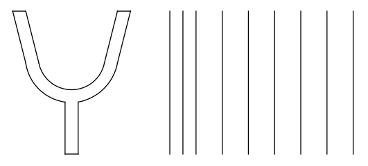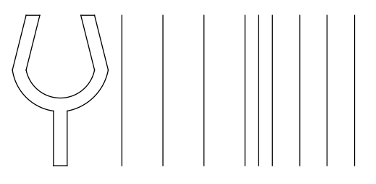Sound waves can be produced using prongs.

Initially, layers of air are in undisturbed positions.

When the prongs are pushed outwards, a region of compression is produced.

When the prongs now move inwards, a region of rarefaction is produced, and the region of compression moves outwards.

In this case, as the prongs now move continuously outwards and inwards, a series of compressions and rarefactions are formed along the layers of air.

Question 1:

Sound wave is an example of:

1. Transverse Wave
2. Longitudinal Wave

Solution:

B) Longitudinal Wave

Explanation:

Sound Wave is a Longitudinal Wave.

## Graph of Sound Waves

### Pressure - Distance Graph

######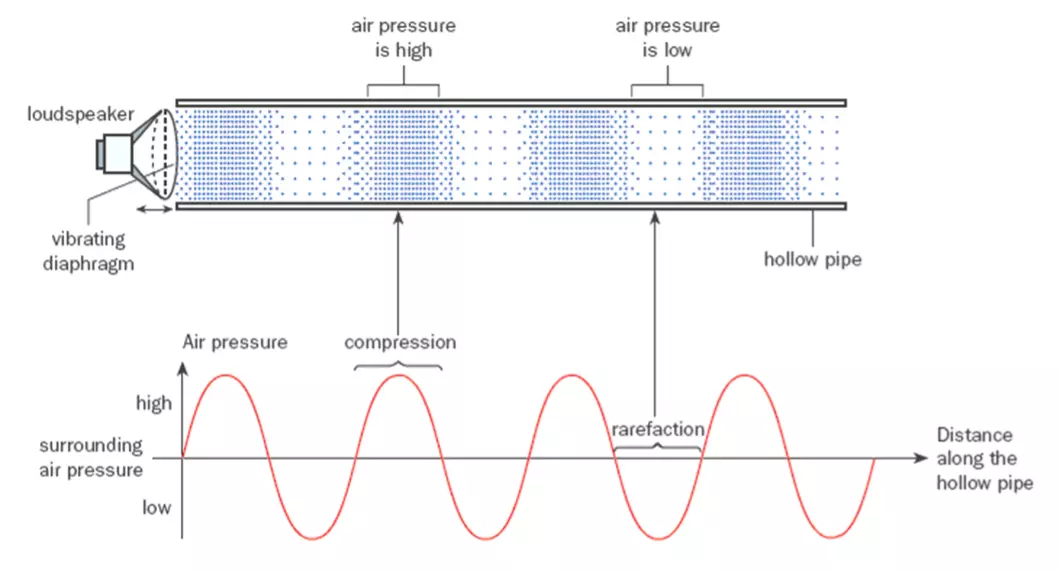Image Credit: mrthou.com

Question 2:

When particles are close together, is it a low pressure area or a high pressure area?

Solution:

Since the particles are close to each other in a given area, therefore the number of particles per unit volume increases and hence it is a high pressure area and hence it is a compression. The centre of compression is at the point of highest pressure, whereas the centre of rarefaction is at the point of lowest pressure.

### Displacement - Distance Graph

######Image Credit: mrthou.com

The centre of compression (C) and centre of rarefaction (R) are where the displacement of particles are zero.

#### Comparison Of Displacement-Distance Graphs And Pressure-Distance Graphs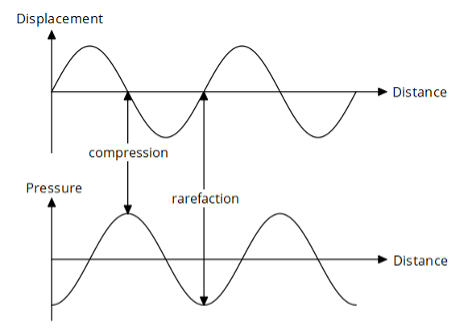When you compare the two graphs, it is helpful to use the centre of compression and rarefaction as reference points.

## Can Sound Be Transmitted Through A Vacuum ?

######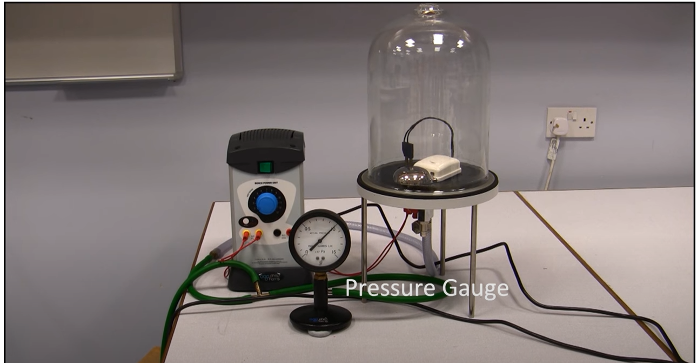Image Credit: youtube.com
• When the electric bell is on and the vacuum pump is off, a sound is heard.
• When the pump is switched on, the sound of the bell becomes softer and softer until we can no longer hear it.

This proves that sound cannot travel through vacuum and needs a medium for transmission.

### Speed Of Sound In Different Medium

Sound travels at different speeds in different media.

Medium Air Water Iron
Approximate speed (m/s) 300
(330 ~ 340)
1500 5000

Order of speed of sound in the 3 states of matter:

$$\text{Gas} \quad\lt\quad \text{Liquid} \quad\lt\quad \text{Solid}$$

In gases, the particles are more loosely packed, and hence the speed of sound is least in gases.

In liquids, the particles are moderately packed and hence the speed of sound in liquid is lower than in solids and faster than in gases.

In solids, the particles are more tightly packed, and hence the speed of sound is fastest in solids.

Nowadays, there are planes that are able to break the sound barrier. Breaking the sound barrier means an object is travelling at a speed that is faster than the speed of sound in air.

Question 2:

The speed of sound in air, water and steel is different. Which of the following sequences is the speed of sound in ascending order?

1. Speed of sound in air, water, steel
2. Speed of sound in steel, water, air
3. Speed of sound in air, steel, water
4. Speed of sound in water, air,  steel

Solution:

A) Speed of sound in air, water, steel

Explanation:

Speed of sound is slowest in the air since it is a gaseous medium, followed by water which is a liquid and fastest in steel which is a solid medium.

Question 3:

During an expedition, NASA astronauts witnessed a huge explosion caused by a meteorite collision. Which of the following is true about the sound produced?

1. It is extremely loud because the surrounding is very quiet.
2. It is extremely loud because sound is amplified in space.
3. It cannot be heard because the frequency is too high
4. It cannot be heard because sound cannot be transmitted in vacuum.

Solution:

D). It cannot be heard because sound cannot be transmitted in vacuum.

Explanation:

It cannot be heard because sound cannot be transmitted in vacuum.
Sound needs particles to travel through a medium as we observed in the Bell-Jar experiment.

Question 4:

Which of the following are always true for sound waves?

1. Sound waves are produced by vibration.
2. Sound waves are longitudinal waves.
3. Sound waves have an approximate speed of 300 m/s.

1. 1 and 1 only
2. 2 and 3 only
3. 1 and 3 only
4. 1, 2 and 3

Solution:

D). 1, 2 and 3

Explanation:

Sound waves are produced by vibrating sources or vibration. Sound waves are also longitudinal waves and they have an approximate speed of 300 m/s in air as observed previously .

Question 5:

An observer hears a thunder 4 seconds after the lightning. Approximately how far is the lightning away from the observer? (Take the speed of sound in air to be 300 m/s).

1. 75 m
2. 600 m
3. 1200 m
4. 2400 m

Solution

C) 1200 m

Explantion:

Since, it is given in the question, the speed of sound is 300 m/s and the time elapsed between the lightning and thunder is 4s when we apply the formula:

$$\textbf{distance } = \textbf{ speed } \times \textbf{ time}$$

Where,

\begin{align} \text{speed} &= 300 \text{ m/s} \quad\& \\[2ex] \text{time} &= 4 \text{ s} \end{align}

Thus,

\begin{align} \text{distance} &= 300 \times 4 \\[2ex] &= 1200 \text{ m} \end{align}

## Conclusion

• How sound is generated by vibrating sources;
• The mechanism by which sound travels, which is through compressions and rarefactions;
• Sound needs a medium to propagate as observed in the Bell- Jar Experiment;
• How sound travels at different speeds in different states of matter..

The article is written to help students studying the Physics coursework in the Secondary 4 grade.

Continue Learning
Electromagnetic Spectrum Sound
Static Electricity D.C. Circuits
Electromagnetic Induction Electromagnetism
Magnetism Current Electricity
Practical ElectricityPrimaryPrimary 1Primary 2Primary 3Primary 4Primary 5Primary 6SecondarySecondary 1Secondary 2Secondary 3Secondary 4Physics
Electromagnetic Spectrum
Sound
Static Electricity
D.C. Circuits
Electromagnetic Induction
Electromagnetism
Magnetism
Current Electricity
Practical ElectricityChemistryMaths